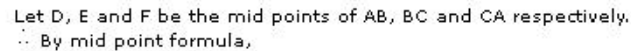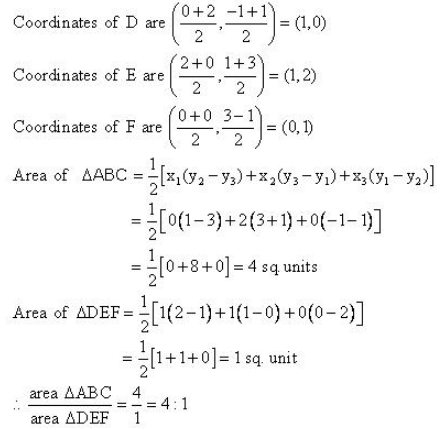# Find the area of the triangle formed by joining the mid points of the sides

Find the area of the triangle formed by joining the midpoints of the sides of a triangle whose vertices are A(0,-1), B(2,1), C(0,3). Find the ratio of this triangle with the given triangle.